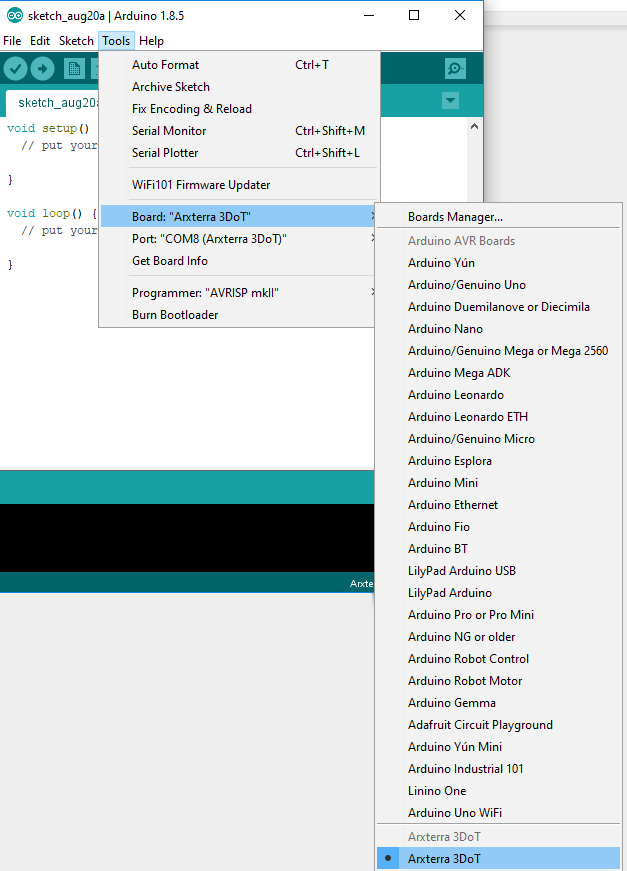# Flood Fill Pathfinding Algorithm

A powerful method of maze solving used for our Micromouse robot.

## How it works

The maze is divided into cells, and the shortest distance from each cell to the goal is calculated. Initially, it is assumed there are no walls in the maze.

Then, as the mouse tries to reach the goal by moving to the neighboring cell with the lowest distance, the distances are updated every time a wall is discovered.

This is repeated, and so the mouse is able to map out the maze and the respective distances to the goal of each cell.

Upon returning to the start, it is simply a matter of connecting the shortest distances to derive a path that leads straight to the goal.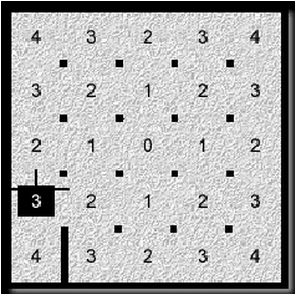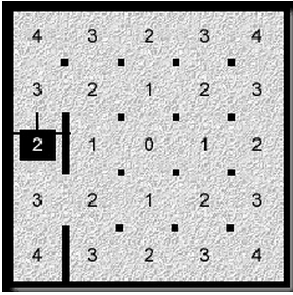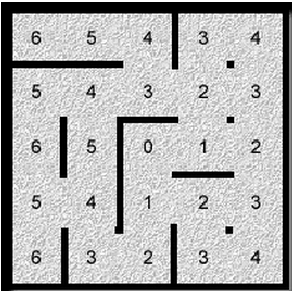## Pseudocode

```create two dimensional array 'distance'
create two dimensional array 'walls'

position = bottom left coordinate of maze

for all elements in walls
element = empty

for all elements in distance
element = shortest distance to goal

while position != goal
if a new wall is discovered
push current coordinate to stack
for all accessible neighboring cells
push neighboring cell coordinates to stack

while !stack.isEmpty
coordinate = pop from stack

if distance != lowest distance of neighbors + 1
distance = lowest distance of neighbors + 1

for all accessible neighboring cells
push neighboring cell coordinates to stack
move to neighbor with lowest distance
```

A stack approach like this is essential, since using recursion will fill up memory much faster on our microcontroller. With a solid understanding of the algorithm and the functions that were going to be needed, it was time to move on to the implementation.

## C++ Implementation

Since the architecture we are using for our mouse is mostly supported by C++ compilers, this was the obvious language to use.

The full code can be viewed on our project's Github page and is free for use.

In order to easily test the algorithm and mouse functions, the code includes a DebugTools class. The printMaze function, for example, prints an ASCII maze including distance values and walls.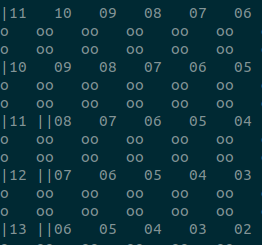Besides the outer edge walls, each wall is displayed twice. This is intended, since walls are also stored in memory twice (one from each neighboring cell).

This function was extremely useful for debugging, and will also be used during live testing of the mouse, as it has capabilities for bluetooth serial communication.